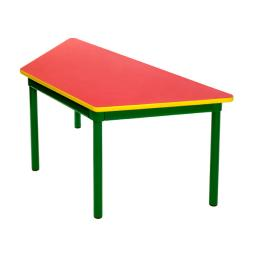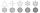# Trapezoid ABCD v2

Trapezoid ABCD has a length of bases in ratio 3:10. The area of triangle ACD is 825 dm2. What is the area of trapezoid ABCD?

S =  1072.5 dm2

### Step-by-step explanation:Did you find an error or inaccuracy? Feel free to write us. Thank you!Tips to related online calculators
Do you have a linear equation or system of equations and looking for its solution? Or do you have a quadratic equation?

#### You need to know the following knowledge to solve this word math problem:

We encourage you to watch this tutorial video on this math problem:

## Related math problems and questions:

• A trapezoid 3A trapezoid ABCD has the bases length of a = 120 mm, c = 86 mm and the area A = 2,575 mm2. Find the height of the trapezoid.
• The ABCDThe ABCD trapezoid has a base length of a = 120mm, c = 86mm and an area of S = 2575 mm2. Calculate the height of the trapezoid.
• 3rd dimensionThe block has a surface of 42 dm2 and its dimensions are 3 dm and 2 dm. What is the third dimension?
• Four prismsQuestion No. 1: The prism has the dimensions a = 2.5 cm, b = 100 mm, c = 12 cm. What is its volume? a) 3000 cm2 b) 300 cm2 c) 3000 cm3 d) 300 cm3 Question No.2: The prism base is a rhombus with a side length of 30 cm and a height of 27 cm. The height of t
• Ratio in trapeziumThe height v and the base a, c in the trapezoid ABCD are in the ratio 1: 6: 3, its content S = 324 square cm. Peak angle B = 35 degrees. Determine the perimeter of the trapezoid
• R TrapeziumRectangular trapezium has bases 21 and 10 and area 92 cm2. What is its perimeter?
• Flowerbed 2Around the square flower bed in a park is sidewalk 2 m wide. The area of ​​this sidewalk is 243 m2. What is the area of the flowerbed?
• Cuboid - ratioFind the volume of a block whose dimensions are in the ratio 2: 3: 4 and the surface is 117 dm2.
• The sides 2The sides of a trapezoid are in the ratio 2:5:8:5. The trapezoid's area is 245. Find the height and the perimeter of the trapezoid.
• The rectangularThe rectangular trapezoid has bases 15 dm and 8 dm long and the length of the inclined arm is 12 dm. How long is the other arm of the trapezoid?
• SquareCalculate the area of the square shape of the isosceles triangle with the arms 50m and the base 60m. How many tiles are used to pave the square if the area of one tile is 25 dm2?
• Isosceles right triangleArea of an isosceles right triangle is 18 dm2. Calculate the length of its base.
• SimilarityThe area of the regular 10-gon is 563 cm2. The area of similar 10-gon is 606 dm2. What is the coefficient of similarity.
• TrapezoidArea of trapezoid is 135 cm2. Sides a, c and height h are in a ratio 6:4:3. How long are a,c and h? Make calculation...
• The circumferenceThe circumference and width of the rectangle are in a ratio of 5: 1. its area is 216cm2. What is its length?
• Trapezoid MO-5-Z8Trapezoid KLMN has bases 12 and 4 cm long. The area of triangle KMN is 9 cm2. What is the area of the trapezoid KLMN?
• Trapezoid 15Area of trapezoid is 266. What value is x if bases b1 is 2x-3, b2 is 2x+1 and height h is x+4